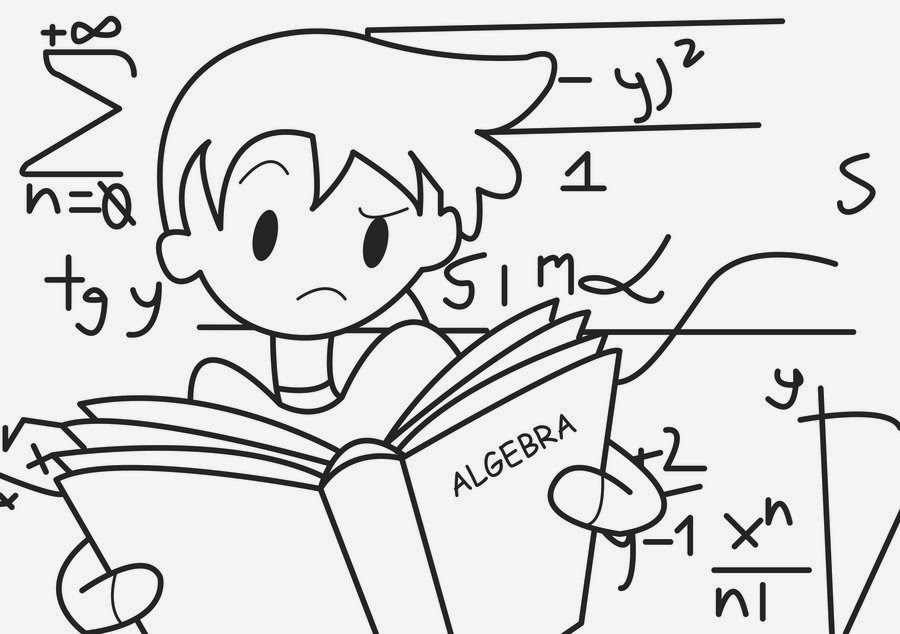## BRANCH OF MATHEMATICS

### Applied Mathematics

In a classroom much of the mathematics we teach is applied mathematics in the sense that it relates directly to life's activities connected with buying, selling, trade, business, consumer applications,weighing, measuring etc. These applications of mathematics to the world around us can be extended to more technical ones.

### Mathematics has helped in analysing motion and in doing so, Newton created the calculus which became known as applied mathematics.

More recently mathematical growth has been in areas such as operational research, linear programming, system analysis, statistics, all involving processes to handle numerical information in an increasingly technologically advanced world. The mathematical ideas we teach in schools develop over many years of study and become associated in our minds with all the applications and illustrations presented to explain them. It is always easier to explain what we can do with a concept in mathematics than to say what it is. A teacher has to answer questions such as "what is the use of this to us this?', or "why do we have to learn this?'If he/she fails to do so there will be many children who will not be able to see the point.

### Pure Mathematics:In pure mathematics we start from certain rules of inference, by which we can infer that if one proposition is true, then some other proposition is also true. These rules oi inference constitute the major part of the principles of formal logic. For instance, we all know the axiom that in real numbers if a > b and b > c, then a > c. Thus, from given propositions we conclude that some other proposition is true. We then take any hypothesis that seems amusing, and deduce its consequences. If our hypothesis is about anything, and not about some (one or more) particular person or thing, then our deductions constitute mathematics.

### Thus, mathematics may be defined as "the subject in which we never know what we are talking about, nor whether what we are saying is me" - Bertrand Russell

These ideas point out the abstract nature of mathematics. Mathematics deals with the application of arbitrary rules in an arbitrary situation which may or may not have significance in the world outside. It is a network of logical relationships. In school mathematics Euclidean geometry is essentially pure mathematics. A set of axioms and postulates are given and from them a body of definitions, theorems and propositions are derived. All pure mathematics is built up by combinations of primitive ideas of logic; its propositions are deduced from the general axioms of logic, such as the syllogism and the other rules of inference.

There is a very thin line dividing pure and applied concepts. On the one hand concepts of pure mathematics are formulated because of the need to apply them and on the other, every discovery or formulation has some application somewhere.

Note: Did you liked the post? Please tell us by your comment below..!!